### Home > CALC > Chapter 1 > Lesson 1.2.4 > Problem1-72

1-72.
1. Sketch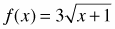on 0 ≤ x ≤ 6 three times, on three different sets of axes. 1-72 HW eTool (Desmos). Homework Help ✎

1. Review your work from problems 1-25 and 1-36. Use a similar process to approximate A(f,0 ≤ x ≤ 6) using:

1. Six left endpoint rectangles.

2. Six right endpoint rectangles.

3. Six trapezoids.

2. Which approximations were over (greater than) estimates of the actual area? Which were under estimates? Explain.

3. Which approximation is more accurate? Explain.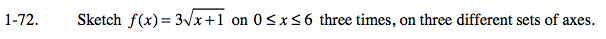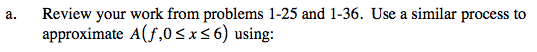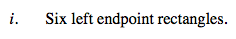The height of each rectangle is 1. The bases can be determined by the function.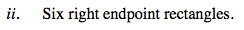The base of each rectangle is 1. The heights can be determined by the function.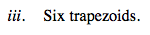The height of each trapezoid is 1. The bases can be determined by the function.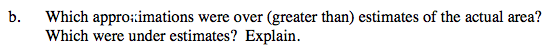f(x) is an increasing and concave down function. Experiment with other increasing functions, and then experiment with other concave down functions. What do you notice about the under/over nature of left-endpoint, right-endpoint and trapezoidal approximations in these more general cases?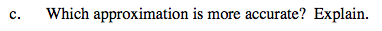Use the eTool below to view the graphs of the function.
Click the link at right for the full version of the eTool: Calc 1-72 HW eTool